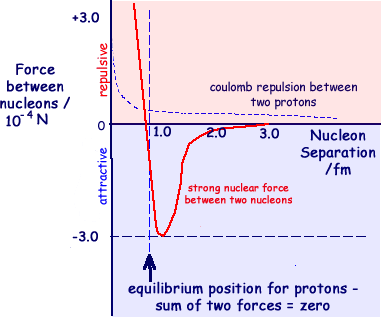# Would higher-mass quarks result in smaller baryons?

• I
• bbbl67

#### bbbl67

Would baryons not made with standard Up or Down quarks exhibit smaller radii than neutrons and protons? I'm thinking like for example how muons have much smaller orbitals than electrons, on the lepton side of things.

•arivero

Last edited:
•mfb, bbbl67, ohwilleke and 1 other person
•bbbl67, ohwilleke and vanhees71
I will talk mostly about the stable octet baryons, because excited baryons can have vastly different radial states. The distance between two quarks in a spin zero state is smaller than the distance in a spin one state because of the spin-spin interaction of quarks. This is why the Delta has a larger radius than the nucleon, and the Lambda has a smaller radius. There is a longer range, Coulomb-like, force between quarks that would give a smaller radius to heavier quarks. That is why the Xis have a smaller radius than the nucleon. The spin-spin interaction is not large for charmed and bottom baryons. That is why they tend to have a smaller radius than the nucleon.

•bbbl67, ohwilleke and malawi_glenn
I will talk mostly about the stable octet baryons, because excited baryons can have vastly different radial states. The distance between two quarks in a spin zero state is smaller than the distance in a spin one state because of the spin-spin interaction of quarks. This is why the Delta has a larger radius than the nucleon, and the Lambda has a smaller radius. There is a longer range, Coulomb-like, force between quarks that would give a smaller radius to heavier quarks. That is why the Xis have a smaller radius than the nucleon. The spin-spin interaction is not large for charmed and bottom baryons. That is why they tend to have a smaller radius than the nucleon.
There is also a repulsive force from the gluons when quarks get too close. Do higher-mass quarks run into that limit if they get too close? Or is the gluon interaction distances different for these other quarks?

I suppose all of those radii would do. What's the difference between them?
the mass radii is a bit "speculative" though there are some peer-reviewed papers trying to calculate it.
it is basically about gravitational interactions.
https://journals.aps.org/prd/pdf/10.1103/PhysRevD.104.054015
https://journals.aps.org/prd/pdf/10.1103/PhysRevD.105.096033
There is also a repulsive force from the gluons when quarks get too close
Source? The gluon exchange is asymptotically free at short rage https://en.wikipedia.org/wiki/Asymptotic_freedom

•dextercioby
the mass radii is a bit "speculative" though there are some peer-reviewed papers trying to calculate it.
it is basically about gravitational interactions.
https://journals.aps.org/prd/pdf/10.1103/PhysRevD.104.054015
https://journals.aps.org/prd/pdf/10.1103/PhysRevD.105.096033
Would this be to figure out if they would be stable inside super-neutron stars?

Source? The gluon exchange is asymptotically free at short rage https://en.wikipedia.org/wiki/Asymptotic_freedom
This graph:This graph:
That is the force between two nucleons, not between two quarks. The force between two nucleons is modeled with meson exchange, not gluon exchange. The repulsive part of the nucleon-nucleon force is due to vector-meson exchange (like ω) iirc.

The force between two quarks become weaker at shorter distances.
In Baryons, you would have quarks with same sign of their charge, so due to their electric replusive force you will at some point not be able to push two quarks closer (in some kind of classical analogy).

Would this be to figure out if they would be stable inside super-neutron stars?
No.

Last edited:
•Btw, speaking of the nucleon-nucleon interaction, it is isopsin dependent. This is why there is only one bound state of two nucleons, the deuteron.

It's nearly isospin symmetric (the explicit violation is due to the mass difference between u and d quarks of a few MeV, i.e., of the same order of magnitude as the violation of chiral symmetry, which is due to the masses of the u and d quarks themselves, also at the order of a few MeV). The fact that there are no bound pp and nn states and that there is no S=0 bound state of np rather indicates that the two-body nucleon-nucleon interaction is spin dependent (but not isospin dependent).

•dextercioby and malawi_glenn
Yeah I f*cked up :)
Of course it is due to spin-dependency, neutrons are identical fermions so their total wavefunction must be antisymmetric (has to form a S=0 state, but there are no bound states with S=0 in the NN-potential)

Last edited:
•vanhees71
That is the force between two nucleons, not between two quarks. The force between two nucleons is modeled with meson exchange, not gluon exchange. The repulsive part of the nucleon-nucleon force is due to vector-meson exchange (like ω) iirc.
Ah, I didn't notice that! I had assumed it was the force within a nucleon, i.e. between quarks.

The force between two quarks become weaker at shorter distances.
In Baryons, you would have quarks with same sign of their charge, so due to their electric replusive force you will at some point not be able to push two quarks closer (in some kind of classical analogy).

No.
Okay, so the EM force becomes important again even within the hadron?

•vanhees71 and malawi_glenn
The force between two quarks become weaker at shorter distances.
The force at moderate distances that affects the mass is Coulombic, like the so called 'Cornell potential'.
The force "becomes weaker" because, for very short distances (or high Q^2), there is 'asymptotic freedom' which softens the $1/r^2$ singularity. The EM force is part of the explanation of mass differences within isomultiplets, but not for the masses asked about in the original question.

•vanhees71